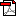# Investigation of Gamma Radiation Shielding Properties of Some Zinc Tellurite Glasses

To cite this article: Issa, S. et al. (2016). Investigation of gamma radiation shielding properties of some zinc tellurite glasses. J. Phys. Sci., 27(3), 97–119, http://dx.doi.org/10.21315/jps2016.27.3.7

ABSTRACT

The mass attenuation coefficient (mm), half-value layer (HVL) and mean free path for xZnO-(100-x) TeO2, where x = 10, 20, 30, and 40 mol.%, have been measured with 0.662, 1.173 and 1.33 MeV photons emitted from 137Cs and 60Co using a 3 x 3 inch NaI(Tl) detector. Some relevant parameters, such as the effective atomic numbers (Zeff) and electron densities (Nel), of the glass samples have also been calculated in the photon energy range of 0.015–15 MeV. Moreover, exposure buildup factors (EBF) were estimated using the five-parameter Geometric Progression (G-P) fitting approximation for penetration depths up to 40 times the mean free path and within the same energy range of 0.015–15 MeV. The measured mass attenuation coefficients were found to agree satisfactorily with the theoretical values obtained using WinXCom. The effective atomic numbers (Zeff) and electron densities (Nel) were found to be the highest for a 40ZnO-60TeO2 glass in the energy range of 0.04–0.2 MeV. The 10ZnO-90TeO2 glass sample had lower values of gamma ray exposure buildup factors in the intermediate energy region. These data on the radiation shielding characteristics of zinc tellurite glasses may be useful for the design of gamma radiation shields.

Download PDFDownload EPUB